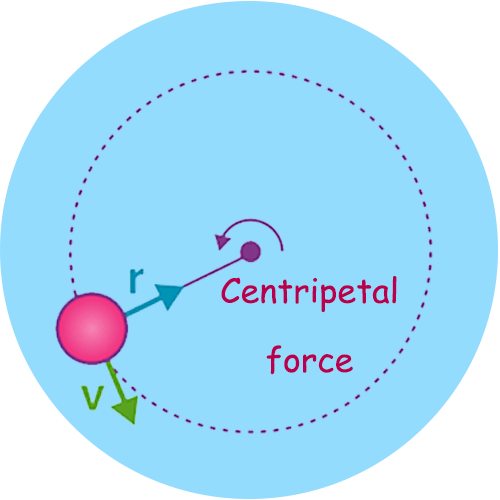# Centripetal Acceleration Calculator

Use our Centripetal Acceleration calculator a free online tool to calculate the centripetal acceleration of the moving body. Enter the values of velocity and radius to calculate. The acceleration, called the centripetal acceleration, points toward the center of the circle.

## Centripetal Acceleration Formula

The magnitude of centripetal acceleration ac= v2 / r### What is Centripetal Acceleration ?

We know from kinematics that acceleration is a change in velocity, either in its magnitude or in its direction, or both. In uniform circular motion, the direction of the velocity changes constantly, so there is always an associated acceleration, even though the magnitude of the velocity might be constant. You experience this acceleration yourself when you turn a corner in your car. (If you hold the wheel steady during a turn and move at constant speed, you are in uniform circular motion.) What you notice is a sideways acceleration because you and the car are changing direction. The sharper the curve and the greater your speed, the more noticeable this acceleration will become.

Centripetal acceleration is the rate of change in velocity of an object that is associated with the change in direction of the velocity.

• Centripetal acceleration is always perpendicular to the velocity.
• Centripetal acceleration always points toward thecenter of the curve.

Example: The friction between the tires and road produces the centripetal acceleration on a level curve.

#### Centripetal Force

The centripetal force may be due to one or more individual forces, such as a normal force and/or a force due to friction.

Fc = m ac